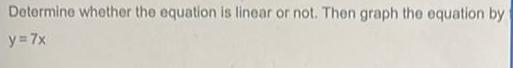Question:

# Determine whether the equation is linear or not. Then graph

Last updated: 7/4/2022Determine whether the equation is linear or not. Then graph the equation by y = 7x